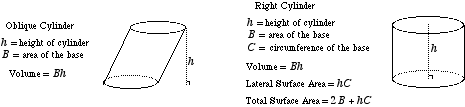index: click on a letter A B C D E F G H I J K L M N O P Q R S T U V W X Y Z A to Z index index: subject areas numbers & symbols sets, logic, proofs geometry algebra trigonometry advanced algebra & pre-calculus calculus advanced topics probability & statistics real world applications multimedia entrieswww.mathwords.com about mathwords website feedback

 Cylinder A three-dimensional geometric figure with parallel congruent bases. The bases can be shaped like any closed plane figure (not necessarily a circle) and must be oriented identically. Note: The word cylinder often refers to a right circular cylinder.See also